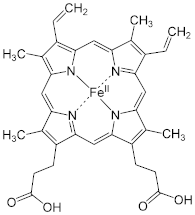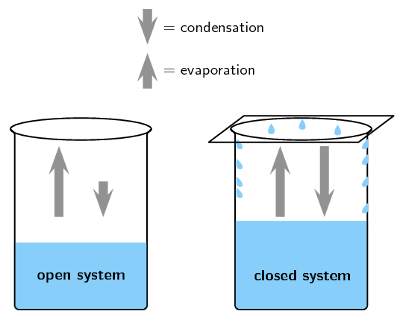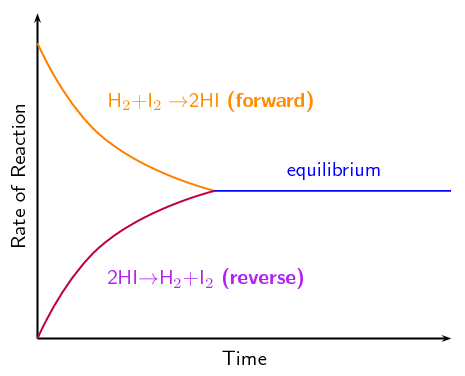Home Practice
For learners and parents For teachers and schools
Textbooks
Full catalogue
Pricing SupportLog in

We think you are located in United States. Is this correct?

# Chapter 8: Chemical equilibrium

In this chapter learners will explore the concept of chemical equilibrium in detail. Before starting this chapter it is essential that the learners have a thorough understanding of rates of reactions and chemical kinetics as covered in Chapter 7. In Grade 11 the definition of equilibrium in physics was covered, it is important that the learners understand the difference between this and a dynamic, chemical equilibrium.

The following topics are covered in this chapter.

• What the term chemical equilibrium means

In this section learners will be introduced to the concept of chemical equilibrium including everyday examples. Covered under this introduction are the concepts of open and closed systems, and the fact that reactions cannot reach equilibrium in an open system. Chemical equilibrium reactions require reversible reactions that can form a dynamic equilibrium, these concepts are also covered here.

• The equilibrium constant

The equilibrium constant is used to determine whether reactants or products are favoured in a reaction at chemical equilibrium. Aside from introducing the meaning of $$\text{K}_{\text{c}}$$ values, this section will require the learners to calculate equilibrium constants from known concentrations, as well as to calculate the concentrations of reactants and products. To do that they should use a RICE table. The acronym makes it easier for learners to remember the information necessary, and the table helps them to organise the information they have and see what information they still need.

It is important that learners have a thorough understanding of equilibrium constants before they begin Chapter 9.

• Le Chatelier's principle

The concept of understanding how a reaction will be affected by an external stress, without actually doing an experiment, is quite abstract and the learners often struggle with it. Lots of examples with different reactions would be a good idea here to make sure they understand the concepts, before you move on to the more advanced interpretations of graphs. The Haber process could be used as an example to make sure they understand the concepts, before you move on to that section.

The informal assessment in this chapter requires the use of concentrated hydrochloric acid. The learners should be properly instructed on the correct use of safety equipment, including safety goggles, gloves and protective clothing. They should also be reminded not to sniff any chemicals as the fumes can be dangerous as well. More information on laboratory procedures as well as safety precautions is provided in Chapter 1 (Science skills). The inital solution at room temperature should be purple. When heated the solution should turn a deep blue, and when cooled it should turn a pink or red colour.

Systems in equilibrium are balanced. For example, if you are running on a treadmill you are in constant motion. However, you are not moving forward or backwards. As fast as you run forward the treadmill is moving you backwards. You are in equilibrium with the treadmill.In the same way chemical reactions can be in equilibrium (the products and reactants are produced at the same rate). We will begin by exploring chemical equilibrium in more detail. Ways of measuring equilibrium and the factors that affect equilibrium will be covered.

## 8.1 What is chemical equilibrium? (ESCND)

To define chemical equilibrium we need to ask some important questions about reactions:

1. Does a reaction always run its full course so that all the reactants are used up?

• When all the reactants in a reaction are used up the reaction is said to have gone to completion. However, in some reactions not all the reactants are used. $$\color{red}{\textbf{Not all reactions go to completion}}$$.
2. Does a reaction always proceed in the same direction or can it be reversed? In other words, does a reaction always proceed reactants $$\to$$ products, or is it possible that a reaction will reverse and go products $$\to$$ reactants?

• Reactions that go to completion are irreversible. However, in some reactions the reactants form products (in a $$\color{red}{\textbf{forward reaction}}$$), and the products can change back into reactants (in a $$\color{red}{\textbf{reverse reaction}}$$).
3. Can a reaction reach a point where reactants are still present, but there does not seem to be any further change taking place in the reaction?

• In all reactions, as the amount of reactant in a reaction decreases the product is formed more slowly. In a reversible reaction, as the amount of product increases the reactant is formed more quickly. Eventually the rate of the forward reaction (reactants $$\to$$ products) equals the rate of the reverse reaction (products $$\to$$ reactants).

At this point there $$\color{red}{\textbf{are still reactants present}}$$ but there does not appear to be any further change taking place. The reaction is said to be in $$\color{blue}{\textbf{chemical}}$$ $$\color{blue}{\textbf{equilibrium}}$$.

Chemical equilibrium is the state of a reversible reaction where the rate of the forward reaction equals the rate of the reverse reaction. While a reaction is in equilibrium the concentration of the reactants and products are constant.

Chemical equilibrium

A reaction is in chemical equilibrium when the rate of the forward reaction equals the rate of the reverse reaction.

There are many examples of chemical equilibrium all around you. One example is a bottle of fizzy cooldrink. In the bottle there is carbon dioxide ($$\text{CO}_{2}$$) dissolved in the liquid. There is also $$\text{CO}_{2}$$ gas in the space between the liquid and the cap. There is a constant movement of $$\text{CO}_{2}$$ from the liquid to the gas phase, and from the gas phase into the liquid. However, if you look at the bottle there does not appear to be any change. The system is in equilibrium.

$\text{CO}_{2}(\text{g}) + \text{H}_{2}\text{O}(\text{l}) \rightleftharpoons \text{H}_{2}\text{CO}_{3}(\text{aq})$

Without chemical equilibrium life as we know it would not be possible. Another example of equilibrium in our everyday lives goes on within our very bodies. Haemoglobin is a macromolecule that transports oxygen around our bodies. Without it we would not survive. The haemoglobin has to be able to take up oxgen, but also to release it and this is done through changes in the chemical equilibrium of this reaction in different places in our bodies.

$\text{haemoglobin(aq)} + 4\text{O}_{2}(\text{g}) \rightleftharpoons \text{haemoglobin}(\text{O}_{2})_{4}(\text{aq})$

Haemoglobin, inside red blood cells in the lungs, bonds with oxygen. This oxyhaemoglobin moves with the red blood cells through the blood stream to cells throughout the body.This video is a good demonstration of how a reaction reaches equilibrium with time.

### Open and closed systems (ESCNF)

The important thing for the students to understand in this experiment is that there is constant movement. Molecules at the surface of the water in the closed beaker are constantly evaporating, and gas molecules are constantly condensing. There is no change in the level of the liquid and the total number molecules in the liquid and the gas state remain constant.

Point out that the level of water in the sealed container also drops initially, before finally remaining constant. This concept will help learners understand how the rate of the forward reaction decreases until equilibrium is reached.

## Liquid-gas phase equilibrium

### Apparatus

• 2 beakers, glass cover

• water

### Method

1. Half-fill two beakers with water and mark the level of the water in each case.

2. Cover one of the beakers with a glass cover.

3. Leave the beakers and, over the course of a day or two, observe how the water levels in the two beakers change. What do you notice? Note: You could speed up this demonstration by placing the two beakers over a Bunsen burner, or in direct sunlight, to heat the water.

### Observations

You should notice that in the beaker that is uncovered, the water level drops more quickly than in the covered beaker. This is because of evaporation. In the beaker that is covered, there is an initial drop in the water level, but after a while evaporation appears to stop and the water level in this beaker is higher than that in the one that is open.### Discussion

In the first beaker, liquid water becomes water vapour as a result of evaporation and the water level drops. A small amount of gas molecules will condense again, but because the gas molecules can escape from the system there is much less condensation than evaporation.

In the second beaker, evaporation also takes place. However, in this case, the vapour comes into contact with the surface of the glass cover and it cools and condenses to form liquid water again. This water is returned to the beaker. Once condensation has begun, the rate at which the water level drops will start to decrease. At some point, the rate of evaporation will be equal to the rate of condensation, and there will be no change in the water level in the beaker. This can be represented as follows:

$$\text{liquid} \rightleftharpoons \text{vapour}$$

In this example, the reaction (in this case, a change in the phase of water) can proceed in either direction. In the forward direction there is a change in phase from liquid to gas, present here as water vapour. A reverse change can also take place, when vapour condenses to form liquid again.

Evaporation is when a substance goes from the liquid phase to the gas phase (it evaporates). Condensation is when a substance goes from the gas phase to the liquid phase (it condenses).

The bottle contains water vapour (gas) and water droplets are condensing on the side of the bottle.An open system is one in which matter or energy can flow into or out of the system. In the liquid-gas demonstration we used, the first beaker was an example of an open system because the beaker could be heated or cooled (a change in energy), and water vapour (the matter) could evaporate from the beaker.

Open system

An open system is one whose borders allow the movement of energy and matter into and out of the system.

A closed system is one in which energy can enter or leave, but matter cannot. The second beaker with the glass cover is an example of a closed system. The beaker can still be heated or cooled, but water vapour cannot leave the system because the glass cover is a barrier. Condensation changes the vapour to liquid and returns it to the beaker. In other words, there is no loss of matter from the system.

Generally, some of the molecules at the surface of liquids and solids are moving into the gas phase. This means that matter is leaving the system. However, this is such a small fraction of the total volume of the liquid or solid that a reaction involving only solids or liquids can be considered a closed system.

It is useful to simplify situations in science by dividing the world into the system we are studying, and the surrounding environment that might influence the reaction, but is not part of it.

Closed system

A closed system is one in which only energy can move into and out of the system. Matter cannot be gained by the system nor lost from the system.

In a closed system it is possible for reactions to be reversible, such as in the demonstration above. In a closed system, it is also possible for a chemical reaction to reach equilibrium.

### Reversible reactions (ESCNG)

Some reactions can take place in two directions. In one direction the reactants combine to form the products. This is called the $$\color{orange}{\text{forward reaction}}$$. In the other direction, the products react to form the reactants again. This is called the $$\color{purple}{\text{reverse reaction}}$$. A special double-headed arrow ( $$\rightleftharpoons$$) is used to show this type of $$\color{blue}{\text{reversible reaction}}$$:

$\text{XY} + \text{Z} \rightleftharpoons \text{X} + \text{YZ}$

A reversible reaction

A reversible reaction is a chemical reaction that can proceed in both the forward and reverse directions. In other words, the reactants and products of the reaction may reverse roles.

Remember that $$\to$$ implies that A goes to B and cannot become A again. $$\rightleftharpoons$$ implies that the reaction is reversible. A can go to B and B can go to A.

So, in the following reversible reaction:

$\overset{{\color{orange}{\textbf{forward}\longrightarrow}}}{\text{H}_{2}\text{(g)} + \text{I}_{2}\text{(g)}} {\color{blue}{\rightleftharpoons}} \underset{\color{purple}{\longleftarrow\textbf{reverse}}}{2\text{HI(g)}}$

$$\color{orange}{{\text{H}}_{2}{\text{(g)}} + {\text{I}}_{2}{\text{(g)}} \to {\text{2HI(g)}}}$$ is the $$\color{orange}{\text{forward}}$$ reaction.

The forward reaction is always taken from the given equation and written: left to right.

$$\color{purple}{{\text{2HI(g)}} \to {\text{H}}_{2}{\text{(g)}} + {\text{I}}_{2}{\text{(g)}}}$$ is the $$\color{purple}{\text{reverse}}$$ reaction.

The reverse reaction is always taken from the given equation and written: right to left.

## Chemical equilibrium

Textbook Exercise 8.1

Which of the following situations describes a closed system?

1. A pot of water (without a lid) heated to $$\text{80}$$$$\text{°C}$$

2. A pot of water (with a lid) heated to $$\text{80}$$$$\text{°C}$$

The water is being heated, therefore there is water in the liquid and the gas phase. The water vapour can leave the pot (system) if there is no lid.

ii) A closed pot of water (with a lid) heated to $$\text{80}$$$$\text{°C}$$

1. $$\text{NaCl}(\text{aq}) + \text{AgNO}_{3}(\text{aq})$$ $$\to$$ $$\text{NaNO}_{3}(\text{aq}) + \text{AlCl}(\text{s})$$

in an open container.

2. $$2\text{CO}(\text{g}) + \text{MoO}_{2}(\text{s})$$ $$\to$$ $$2\text{CO}_{2}(\text{g}) + \text{Mo}(\text{s})$$

in an open container.

Assume that none of the solid or liquid reactants or products go into the gas phase.

i) $$\text{NaCl}(\text{aq}) + \text{AgNO}_{3}(\text{aq})$$ $$\to$$ $$\text{NaNO}_{3}(\text{aq}) + \text{AlCl}(\text{s})$$

For the reaction: $$\text{A} + \text{B}$$ $$\rightleftharpoons$$ $$\text{C} + \text{D}$$

Write down the forward reaction

$$\text{A} + \text{B}$$ $$\rightarrow$$ $$\text{C} + \text{D}$$

Write down the reverse reaction

$$\text{C} + \text{D}$$ $$\rightarrow$$ $$\text{A} + \text{B}$$

This reaction is said to be ....... (fill in the missing word)

This reaction is said to be reversible

### Dynamic equilibrium (ESCNH)

We will use the same reversible reaction that we used in an earlier example:

$\color{blue}{\text{H}_{2}{\text{(g)}} + {\text{I}}_{2}{\text{(g)}} \rightleftharpoons {\text{2HI(g)}}}$

The $$\color{orange}{\text{forward}}$$ reaction is: $$\color{orange}{\text{H}_{2}{\text{(g)}} + {\text{I}}_{2}{\text{(g)}} \to {\text{2HI(g)}}}$$

The $$\color{purple}{\text{reverse}}$$ reaction is: $$\color{purple}{\text{2HI(g)} \to {\text{H}}_{2}{\text{(g)}} + {\text{I}}_{2}{\text{(g)}}}$$

When the rate of the forward reaction and the rate of the reverse reaction are equal, the system is said to be in $$\color{blue}{\text{equilibrium}}$$. Figure 8.1 shows this. Initially (time = $$\text{0}$$), the rate of the $$\color{orange}{\text{forward reaction}}$$ is high (fast). With time, the rate of the $$\color{orange}{\text{forward reaction decreases}}$$. As the reaction gets closer to equilibrium the rate of decrease levels out until the forward reaction has a constant rate.

Initially the rate of the $$\color{purple}{\text{reverse reaction}}$$ is low (slow). As the reaction proceeds with time, the rate of the $$\color{purple}{\text{reverse reaction increases}}$$. As the reaction progresses the rate of increase levels out until the reverse reaction has a constant rate.

At this point the forward and reverse reaction rates are equal and this is called $$\color{blue}{\textbf{equilibrium}}$$.Figure 8.1: The change in rate of $$\color{orange}{\text{forward}}$$ and $$\color{purple}{\text{reverse}}$$ reactions in a closed system.

Although it is not always possible to observe any macroscopic changes, this does not mean that the reaction has stopped. The forward and reverse reactions continue to take place and so microscopic changes still occur in the system. This state is called dynamic equilibrium.

Dynamic equilibrium

There is a dynamic equilibrium in a reversible reaction when the rate of the forward reaction equals the rate of the reverse reaction. The amounts of reactants and products remain constant.

In the liquid-gas phase equilibrium demonstration, dynamic equilibrium was reached when there was no observable change in the level of the water in the second beaker even though evaporation and condensation continued to take place.